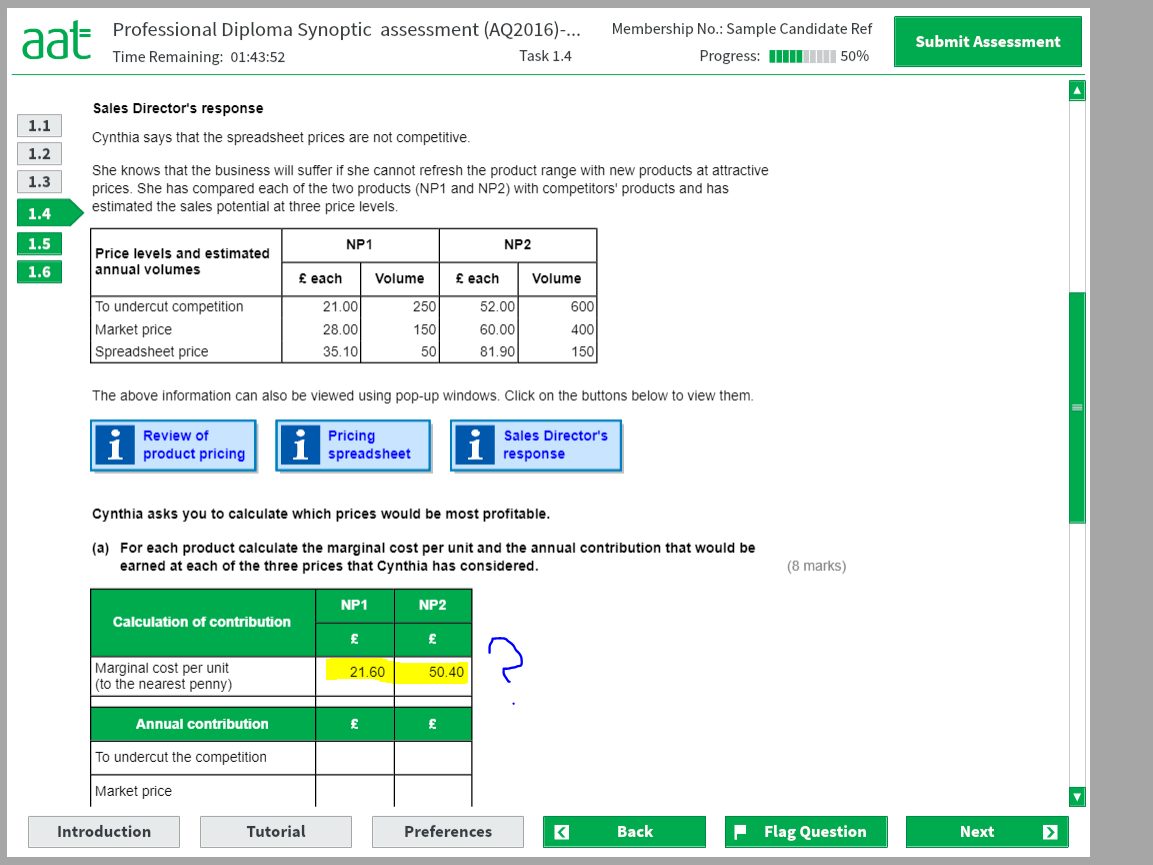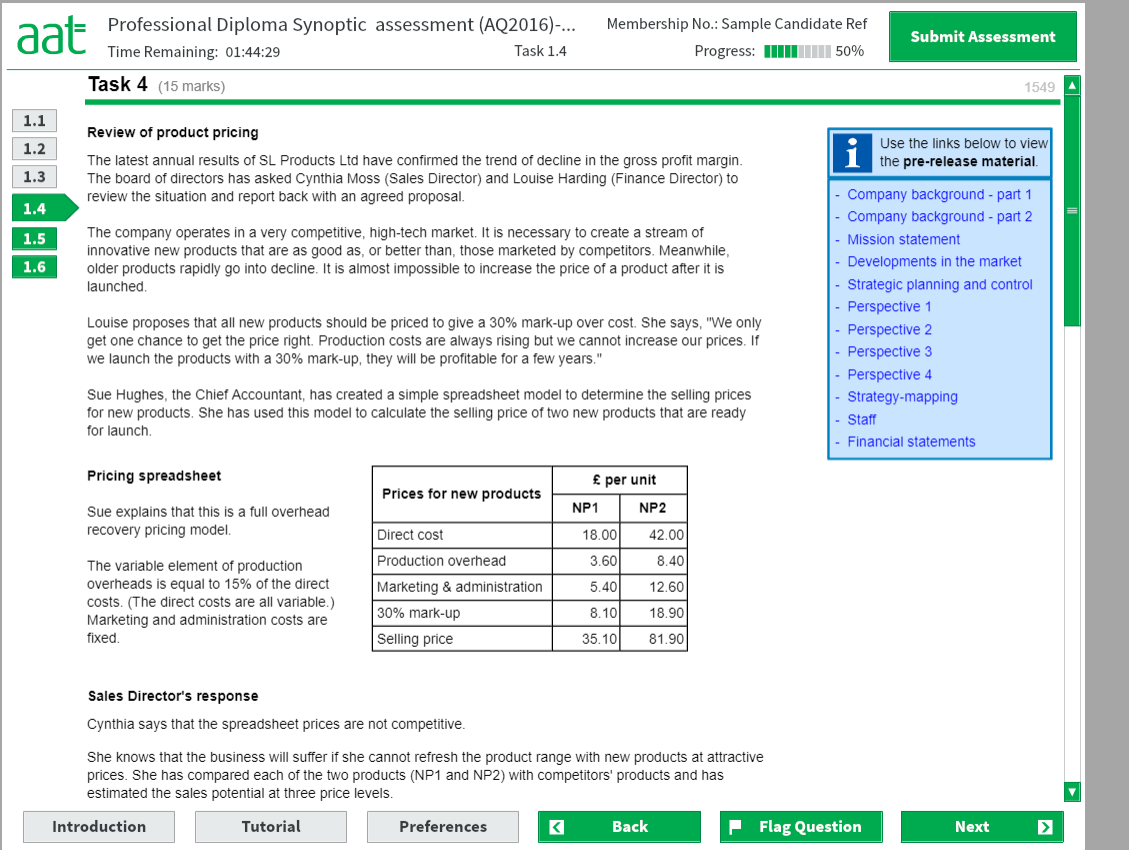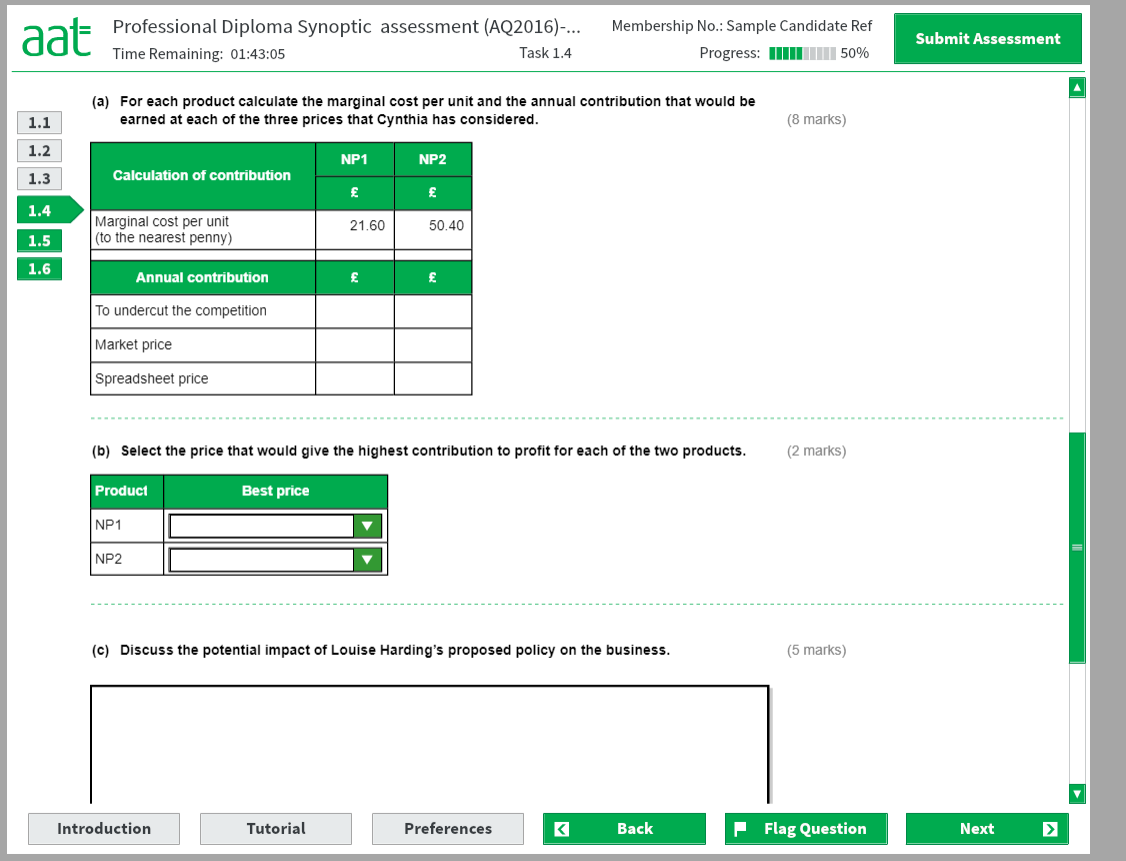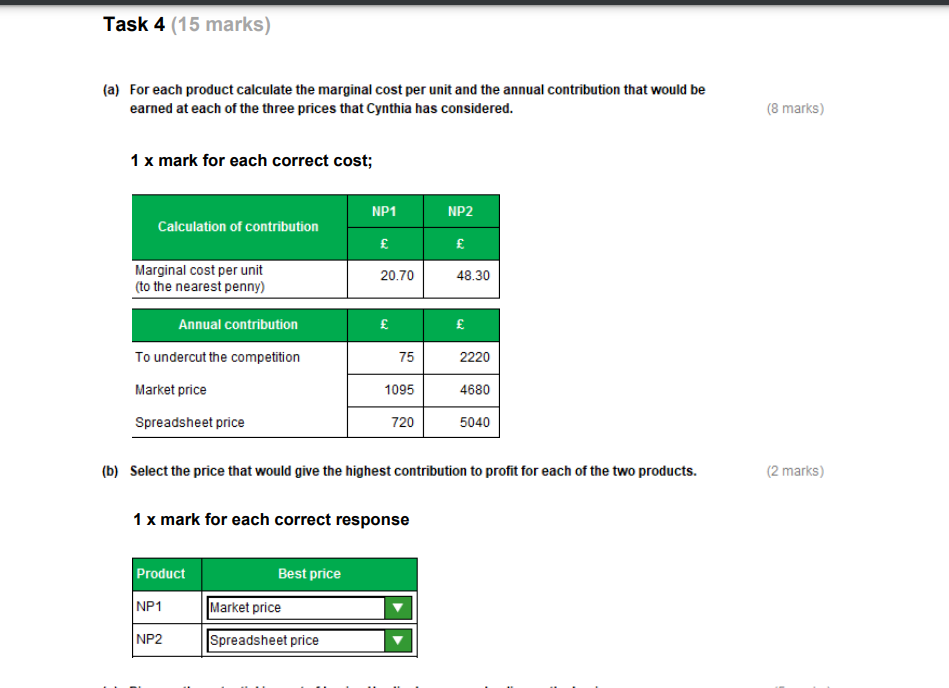# Marginal Costing - Level 4 synoptic sample assesment task 4

Can someone please explain how to calculate the marginal cost from the given info?
And then how to answer the rest, if poss?
Thank you• Almost there!

Just look at the note to the right of the pricing spreadsheet and it states only 15% of the production overheads are direct costs. Production variable cost: NP1 this is 54p and for NP2 it is £1.26.
Marginal costs are therefore £18.54 for NP1, and £43.26 for NP2.

You then calculate the contribution per unit for each scenario (Undercut/Market/Spreadsheet) and then multiply by expected annual demand.

So as example, NP1 at the price to undercut. Contribution per unit is £2.46 (£21.00 - £18.54). Annual contribution is then £615 (£2.46 x 250).

The calculation part is sorted!

Then just pick best scenario for NP1 and NP2 for (b) and then discuss in (c)

• This is also what I thought but the model answers give a different result• Evil Calculator's calculation is wrong.

Marginal cost per unit is basically the variable cost

It says the variable element of the production overheads is equal to 15% of the direct cost, therefore £2.70 (15% of £18) for NP1 and £6.30 (15% of £42) for NP2

We then add on the direct cost for each to give us the marginal cost per unit of £20.70 (18+2.70) and £48.30 (42+6.30) for NP1 and NP2 respectively

The next part of the question is concerned with contribution which is the selling price-variable costs (i.e the marginal cost calculation above). To give annual contribution we would then multiply by the volume

NP1 calculations:
To undercut competition: (21-20.70) x 250 = 75
Market price: (28-20.70) x 150 = 1,095 HIGHEST CONTRIBUTION
Spreadsheet price: (35.10-20.70) x 50 = 720

NP2 calculations:
To undercut competition: (52-48.30) x 600 = 2,200
Market price: (60-48.30) x 400 = 4,680
Spreadsheet price: (81.90-48.30) x 150 = 5,040 HIGHEST CONTRIBUTION

Then in part c, you have to explain the impact on the business of using Louise's policy. You could discuss things like:
Her policy means that the products will be profitable no matter the cost, because the selling price will be set based on 30% mark-up on cost.
However, SLP operates in a competitive market, therefore needs to offer competitive prices. Setting selling prices too high, will mean customers will go to our competitors, meaning we would only be able to sell in small amounts, which increases the risk of being unable to recover our fixed costs

Hopefully that makes sense
AAT Level 2: Distinction
BTRN (96%), BKCL (99%), ELCO (95%), UACS (98%), FSYA (96%)

AAT Level 3: Distinction
AVBK (94%), FAPR (93%), MMAC (99%), AVSY (93%), IDRX (94%)

AAT Level 4: Ongoing
MABU (90%), MDCL (86%), FSLC (94%)